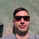7252 lượt xem
7252
original script by /u/SandroTurriate/ - I just made some small changes.

Vwap + standard deviation bands. Good for reversal trading among other things. Used intraday.
Very useful when price is ranging.

I added the option to fill the spaces between the deviation lines with color and also the option to add some extra bands. That's about it. Color/length/style etc is customizable.

```study("VWAP Stdev Bands v2 Mod", overlay=true)
devUp1 = input(1.28, title="Stdev above (1)")
devDn1 = input(1.28, title="Stdev below (1)")

devUp2 = input(2.01, title="Stdev above (2)")
devDn2 = input(2.01, title="Stdev below (2)")

devUp3 = input(2.51, title="Stdev above (3)")
devDn3 = input(2.51, title="Stdev below (3)")

devUp4 = input(3.09, title="Stdev above (4)")
devDn4 = input(3.09, title="Stdev below (4)")

devUp5 = input(4.01, title="Stdev above (5)")
devDn5 = input(4.01, title="Stdev below (5)")

showDv2 = input(true, type=bool, title="Show second group of bands?")
showDv3 = input(true, type=bool, title="Show third group of bands?")
showDv4 = input(false, type=bool, title="Show fourth group of bands?")
showDv5 = input(false, type=bool, title="Show fifth group of bands?")

showPrevVWAP = input(false, type=bool, title="Show previous VWAP close")

start = security(tickerid, "D", time)

newSession = iff(change(start), 1, 0)

vwapsum = iff(newSession, hl2*volume, vwapsum+hl2*volume)
volumesum = iff(newSession, volume, volumesum+volume)
v2sum = iff(newSession, volume*hl2*hl2, v2sum+volume*hl2*hl2)
myvwap = vwapsum/volumesum
dev = sqrt(max(v2sum/volumesum - myvwap*myvwap, 0))

A=plot(myvwap,style=circles, title="VWAP", color=black)
U1=plot(myvwap + devUp1 * dev,style=circles, title="VWAP Upper", color=gray)
D1=plot(myvwap - devDn1 * dev, style=circles, title="VWAP Lower", color=gray)

U2=plot(showDv2 ? myvwap + devUp2 * dev : na, color=red, title="VWAP Upper (2)")
D2=plot(showDv2 ? myvwap - devDn2 * dev : na, color=green, title="VWAP Lower (2)")

U3=plot(showDv3 ? myvwap + devUp3 * dev : na, title="VWAP Upper (3)", color=red)
D3=plot(showDv3 ? myvwap - devDn3 * dev : na, title="VWAP Lower (3)", color=green)

U4=plot(showDv4 ? myvwap + devUp4 * dev : na, title="VWAP Upper (4)", color=red)
D4=plot(showDv4 ? myvwap - devDn4 * dev : na, title="VWAP Lower (4)", color=green)

U5=plot(showDv5 ? myvwap + devUp5 * dev : na, title="VWAP Upper (5)", color=red)
D5=plot(showDv5 ? myvwap - devDn5 * dev : na, title="VWAP Lower (5)", color=green)
prevwap = iff(newSession, myvwap, prevwap)
plot(showPrevVWAP ? prevwap : na, style=circles, color=close > prevwap ? green : red)

fill(U1, U2, color=red, transp=90, title="Over Bought Fill 1")
fill(D1, D2, color=green, transp=90, title="Over Sold Fill 1")
fill(U2, U3, color=red, transp=90, title="Over Bought Fill 2")
fill(D2, D3, color=green, transp=90, title="Over Sold Fill 2")
fill(U3, U4, color=red, transp=90, title="Over Bought Fill 3")
fill(D3, D4, color=green, transp=90, title="Over Sold Fill 3")
fill(U4, U5, color=red, transp=90, title="Over Bought Fill 4")
fill(D4, D5, color=green, transp=90, title="Over Sold Fill 4")
fill(A, U1, color=gray, transp=90, title="Middle Fill Up")
fill(A, D1, color=gray, transp=90, title="Middle Fill Down")
```What is the best settings to use on higher timeframes? Namely W1, D1, H4 and H1?
Phản hồiCan i use it on 30min candels and seeing the weekly vwap deviations ?
Phản hồi